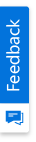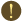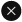We use cookies to give you the best experience on our website. If you continue to browse, then you agree to our privacy policy and cookie policy.Unfortunately, activation email could not send to your email. Please try again.# Blazor Combination Chart - Visualize and Compare Different Data Sets

## Overview

The Blazor Combination Chart combines two or more chart types into a single chart to compare different data sets. Supports zooming, panning, tooltip, trackball, and selection.

## Combination of line, area, and column chart

Create multiple series of different types in the same chart. Legend and tooltip for the series make it more readable.

## Pareto chart

Blazor Pareto Chart is a combination of line and column charts. The column represents data in the descending order, whereas the line chart represents the cumulative value of the data.

## Combination of Candle with Column/Line

You can combine Candle with line or column chart to show additional parameters in financial analysis. For example, volume of the stock can be viewed with the help of column chart.

## Blazor Combination Chart Code Example

Easily get started with Blazor Combination Chart using a few simple lines of C# code, as demonstrated below,

```@using Syncfusion.Blazor.Charts

<SfChart>
<ChartPrimaryXAxis ValueType="Syncfusion.Blazor.Charts.ValueType.Category"></ChartPrimaryXAxis>

<ChartSeriesCollection>
<ChartSeries DataSource="@SalesReports" Name="Private Consumption" XName="X" YName="Y" Type="ChartSeriesType.StackingColumn">
</ChartSeries>
<ChartSeries DataSource="@SalesReports" Name="Government Consumption" XName="X" YName="Y1" Type="ChartSeriesType.StackingColumn">
</ChartSeries>
<ChartSeries DataSource="@SalesReports" Name="Investment" XName="X" YName="Y2" Type="ChartSeriesType.StackingColumn">
</ChartSeries>
<ChartSeries DataSource="@SalesReports" Name="Net Foreign Trade" XName="X" YName="Y3" Type="ChartSeriesType.StackingColumn">
</ChartSeries>
<ChartSeries DataSource="@SalesReports" Name="GDP" XName="X" YName="Y4" Width="2" Opacity="0.6" Type="ChartSeriesType.Line">
<ChartMarker Visible="true" Height="10" Width="10"></ChartMarker>
</ChartSeries>
</ChartSeriesCollection>
</SfChart>

@code{
public class ChartData
{
public string X { get; set; }
public double Y { get; set; }
public double Y1 { get; set; }
public double Y2 { get; set; }
public double Y3 { get; set; }
public double Y4 { get; set; }
}
public List<ChartData> SalesReports = new List<ChartData>
{
new ChartData { X= "2005", Y= 1.2, Y1= 0.5, Y2= 0.7, Y3= -0.8, Y4= 1.5 },
new ChartData { X= "2006", Y= 1, Y1= 0.5, Y2= 1.4, Y3= 0, Y4= 2.3 },
new ChartData { X= "2007", Y= 1, Y1= 0.5, Y2= 1.5, Y3= -1, Y4= 2 },
new ChartData { X= "2008", Y= 0.25, Y1= 0.35, Y2= 0.35, Y3= -.35, Y4= 0.1 },
new ChartData { X= "2009", Y= 0.1, Y1= 0.9, Y2= -2.7, Y3= -0.3, Y4= -2.7 },
new ChartData { X= "2010", Y= 1, Y1= 0.5, Y2= 0.5, Y3= -0.5, Y4= 1.8 },
new ChartData { X= "2011", Y= 0.1, Y1= 0.25, Y2= 0.25, Y3= 0, Y4= 2 },
new ChartData { X= "2012", Y= -0.25, Y1= -0.5, Y2= -0.1, Y3= -0.4, Y4= 0.4 },
new ChartData { X= "2013", Y= 0.25, Y1= 0.5, Y2= -0.3, Y3= 0, Y4= 0.9 },
new ChartData { X= "2014", Y= 0.6, Y1= 0.6, Y2= -0.6, Y3= -0.6, Y4= 0.4 },
new ChartData { X= "2015", Y= 0.9, Y1= 0.5, Y2= 0, Y3= -0.3, Y4= 1.3 }
};
}```

## Not sure how to create your first Blazor Combination Chart? Our documentation can help.

### Blazor Components – 80+ UI and DataViz Components

ALL COMPONENTS
DOCUMENT PROCESSING LIBRARIESYou are using an outdated version of Internet Explorer that may not display all features of this and other websites. Upgrade to Internet Explorer 8 or newer for a better experience.